SBOS724A September   2015  – June 2022

PRODUCTION DATA

1. Features
2. Applications
3. Description
4. Revision History
5. Device Comparison Table
6. Pin Configuration and Functions
7. Specifications
8. Detailed Description
1. 8.1 Overview
2. 8.2 Functional Block Diagram
3. 8.3 Feature Description
4. 8.4 Device Functional Modes
9. Applications and Implementation
1. 9.1 Application Information
2. 9.2 Typical Application
1. 9.2.1 Headphone Amplifier Circuit Configuration
10. 10Power Supply Recommendations
11. 11Layout
12. 12Device and Documentation Support
1. 12.1 Device Support
1. 12.1.1 Development Support
2. 12.2 Documentation Support
4. 12.4 Support Resources
6. 12.6 Electrostatic Discharge Caution
7. 12.7 Glossary
13. 13Mechanical, Packaging, and Orderable Information

• D|8
• DRG|8
• DRG|8

## 7.6 Typical Characteristics

at VS = ±18 V, VCM = VS / 2, RLOAD = 10 kΩ connected to VS / 2, and CL = 100 pF (unless otherwise noted)

Table 7-1 List of Typical Characteristics
DESCRIPTION FIGURE
Offset Voltage Production Distribution Figure 7-1
Offset Voltage Drift Distribution Figure 7-2
Offset Voltage vs Temperature (VS = ±18 V) Figure 7-3
Offset Voltage vs Common-Mode Voltage (VS = ±18 V) Figure 7-4
Offset Voltage vs Common-Mode Voltage (Upper Stage) Figure 7-5
Offset Voltage vs Power Supply Figure 7-6
Input Bias Current vs Common-Mode Voltage Figure 7-7
Input Bias Current vs Temperature Figure 7-8
Output Voltage Swing vs Output Current (Maximum Supply) Figure 7-9
CMRR and PSRR vs Frequency (Referred-to-Input) Figure 7-10
CMRR vs Temperature Figure 7-11
PSRR vs Temperature Figure 7-12
0.1-Hz to 10-Hz Noise Figure 7-13
Input Voltage Noise Spectral Density vs Frequency Figure 7-14
THD+N Ratio vs Frequency Figure 7-15
THD+N vs Output Amplitude Figure 7-16
THD+N vs Frequency Figure 7-17
THD+N vs Amplitude Figure 7-18
Quiescent Current vs Temperature Figure 7-19
Quiescent Current vs Supply Voltage Figure 7-20
Open-Loop Gain and Phase vs Frequency Figure 7-21
Closed-Loop Gain vs Frequency Figure 7-22
Open-Loop Gain vs Temperature Figure 7-23
Open-Loop Output Impedance vs Frequency Figure 7-24
Small-Signal Overshoot vs Capacitive Load (100-mV Output Step) Figure 7-25, Figure 7-26
Positive Overload Recovery Figure 7-27, Figure 7-28
Negative Overload Recovery Figure 7-29, Figure 7-30
Small-Signal Step Response (10 mV, G = –1) Figure 7-31
Small-Signal Step Response (10 mV, G = 1) Figure 7-32
Small-Signal Step Response (100 mV, G = –1) Figure 7-33
Small-Signal Step Response (100 mV, G = 1) Figure 7-34
Large-Signal Step Response (10 V, G = –1) Figure 7-35
Large-Signal Step Response (10 V, G = 1) Figure 7-36
Large-Signal Settling Time (10-V Positive Step) Figure 7-37
Large-Signal Settling Time (10-V Negative Step) Figure 7-38
No Phase Reversal Figure 7-39
Short-Circuit Current vs Temperature Figure 7-40
Maximum Output Voltage vs Frequency Figure 7-41
EMIRR vs Frequency Figure 7-42
Channel Separation vs Frequency Figure 7-43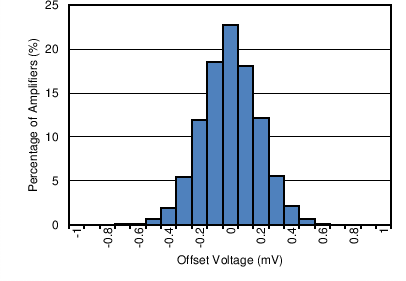Distribution taken from 5185 amplifiers
Figure 7-1 Offset Voltage Production Distribution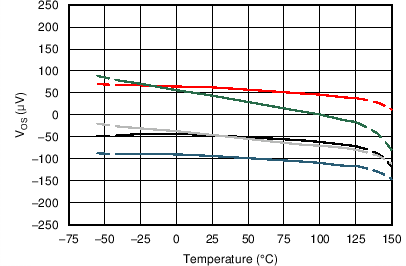5 typical units shown, VS = ±18 V
Figure 7-3 Offset Voltage vs Temperature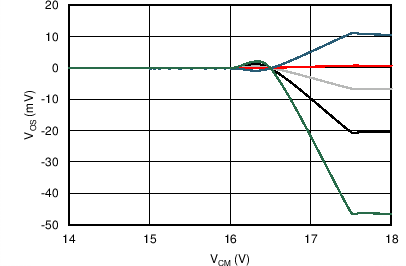5 typical units shown, VS = ±18 V
Figure 7-5 Offset Voltage vs Common-Mode Voltage (Upper Stage)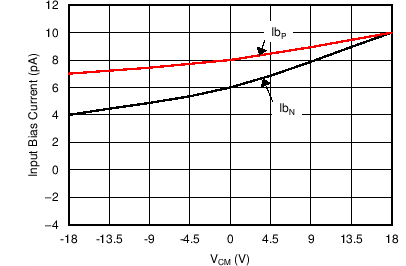TA = 25°C
Figure 7-7 Input Bias Current vs Common-Mode Voltage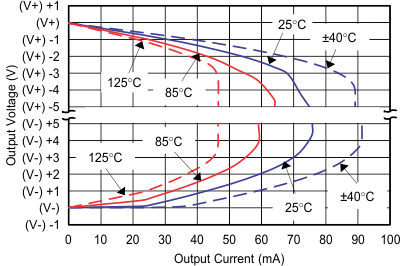Figure 7-9 Output Voltage Swing vs Output Current (Maximum Supply)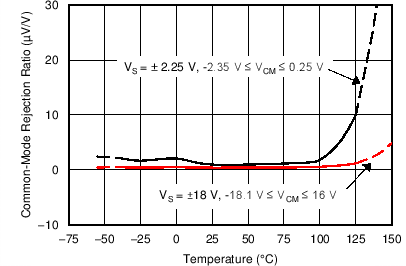Figure 7-11 CMRR vs Temperature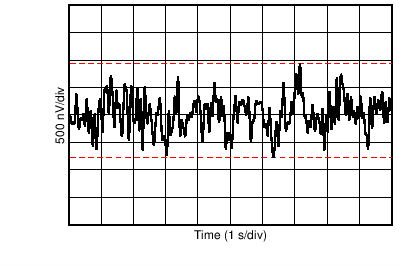Peak-to-peak noise = 1.70 µVPP
Figure 7-13 0.1-Hz to 10-Hz Noise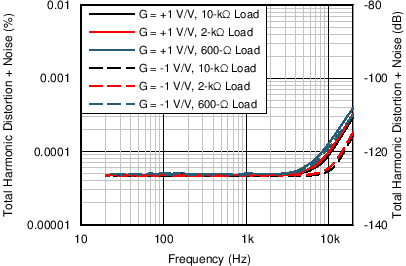VOUT = 3.5 VRMS, BW = 50 kHz
Figure 7-15 THD+N Ratio vs Frequency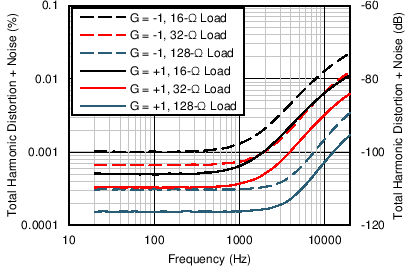POUT = 10 mW, BW = 80 kHz, VS = ±5 V
Figure 7-17 THD+N vs FrequencyFigure 7-19 Quiescent Current vs TemperatureFigure 7-21 Open-Loop Gain and Phase vs Frequency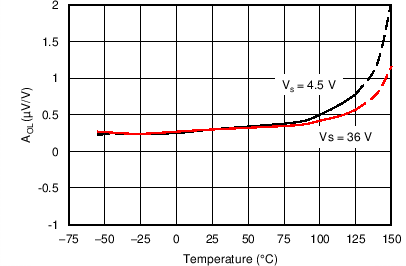RL = 10 kΩ
Figure 7-23 Open-Loop Gain vs Temperature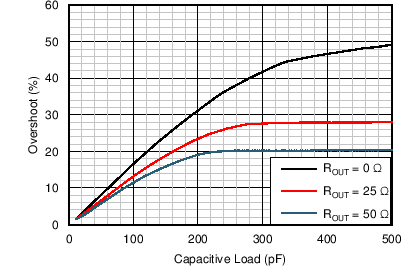G = –1
Figure 7-25 Small-Signal Overshoot vs Capacitive Load (100‑mV Output Step)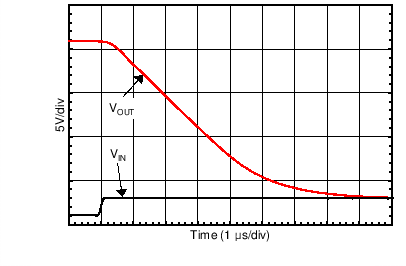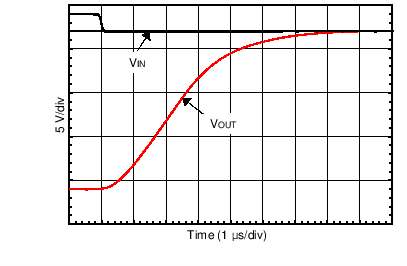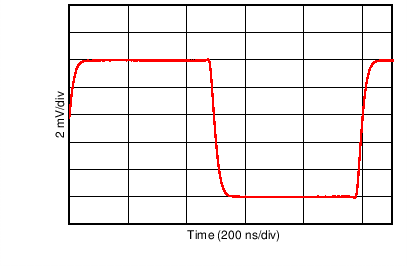10 mV, G = –1, RL = 1 kΩ, CL = 10 pF
Figure 7-31 Small-Signal Step Response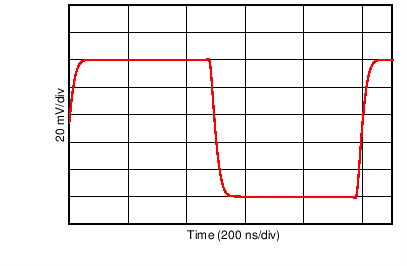100 mV, G = –1, RL = 1 kΩ, CL = 10 pF
Figure 7-33 Small-Signal Step Response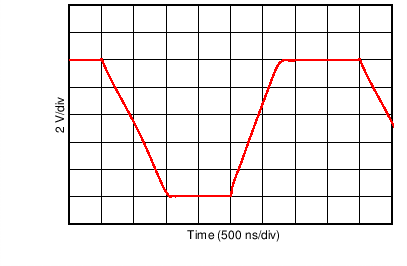10 V, G = –1, RL = 1 kΩ, CL = 10 pF
Figure 7-35 Large-Signal Step Response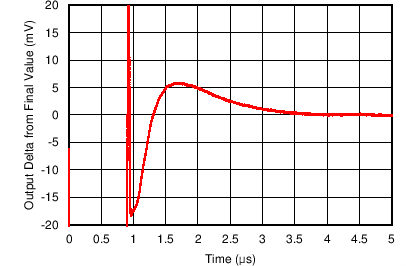G = 1, CL = 10 pF, 0.1% settling = ±10 mV
Figure 7-37 Large-Signal Settling Time (10‑V Positive Step)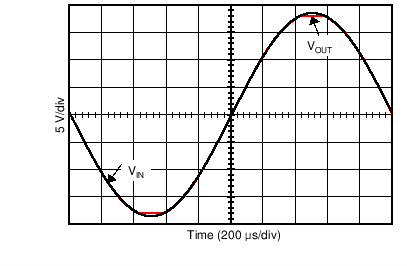Figure 7-39 No Phase Reversal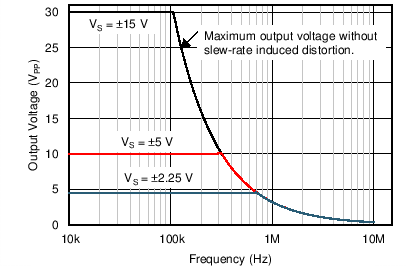Figure 7-41 Maximum Output Voltage vs Frequency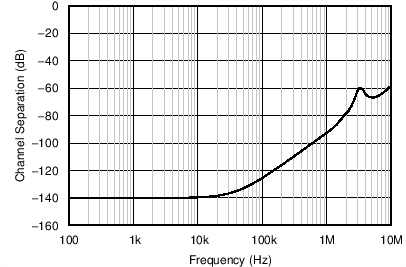Figure 7-43 Channel Separation vs Frequency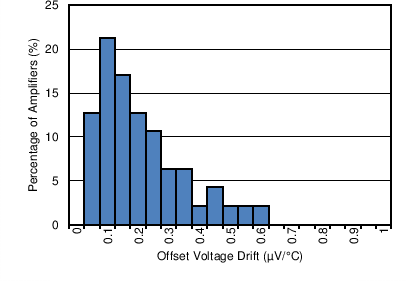Distribution taken from 47 amplifiers, TA = –40°C to +125°C
Figure 7-2 Offset Voltage Drift Production Distribution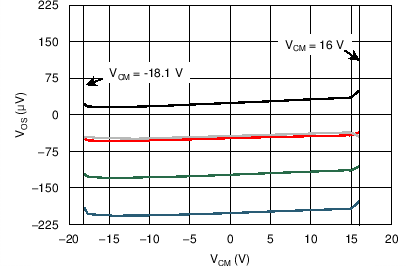5 typical units shown, VS = ±18 V
Figure 7-4 Offset Voltage vs Common-Mode Voltage5 typical units shown, VS = ±2.25 V to ±18 V
Figure 7-6 Offset Voltage vs Power Supply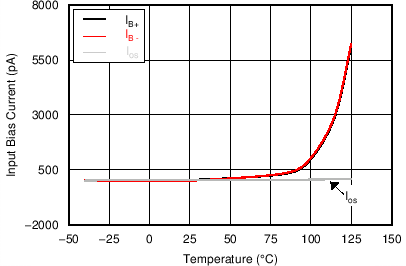Figure 7-8 Input Bias Current vs Temperature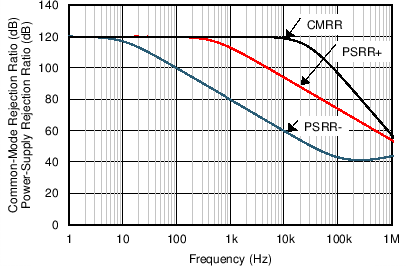Figure 7-10 CMRR and PSRR vs Frequency (Referred-to-Input)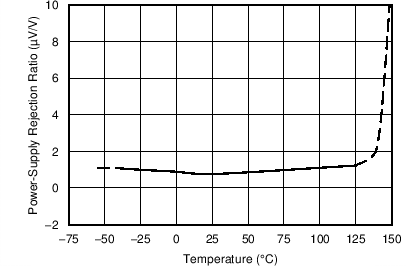Figure 7-12 PSRR vs Temperature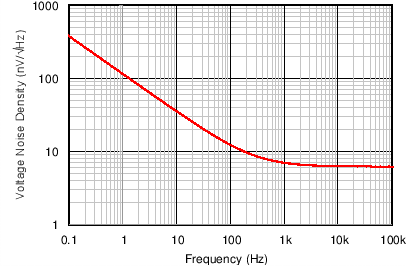Figure 7-14 Input Voltage Noise Spectral Density vs Frequency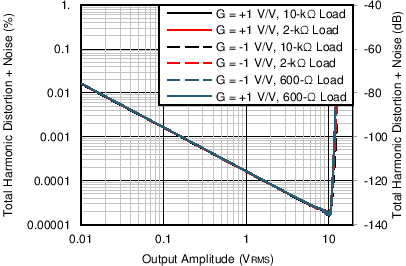f = 1 kHz, BW = 80 kHz
Figure 7-16 THD+N vs Output Amplitude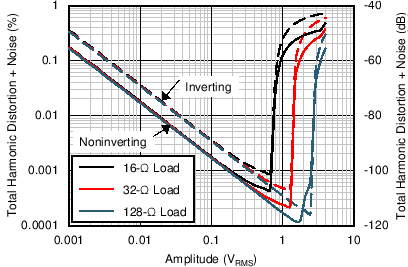f = 1 kHz, BW = 80 kHz, VS = ±5 V
Figure 7-18 THD+N vs AmplitudeFigure 7-20 Quiescent Current vs Supply Voltage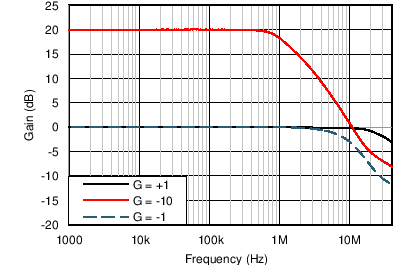Figure 7-22 Closed-Loop Gain vs Frequency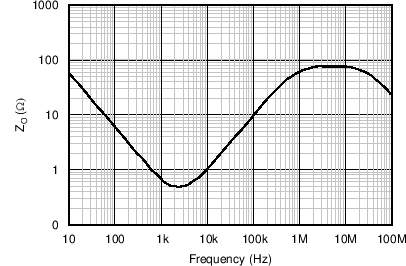Figure 7-24 Open-Loop Output Impedance vs Frequency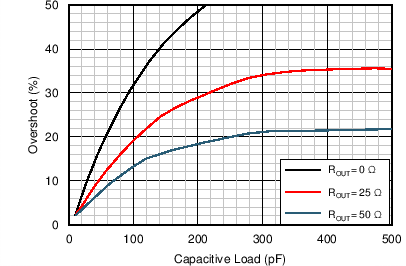G = 1
Figure 7-26 Small-Signal Overshoot vs Capacitive Load (100‑mV Output Step)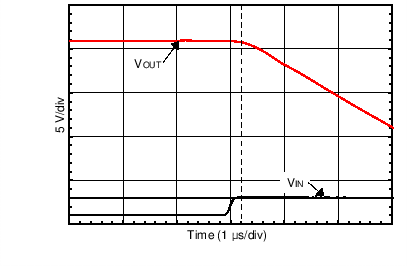Figure 7-28 Positive Overload Recovery (Zoomed In)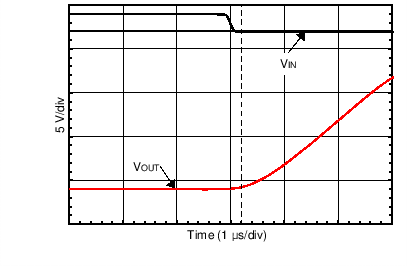Figure 7-30 Negative Overload Recovery (Zoomed In)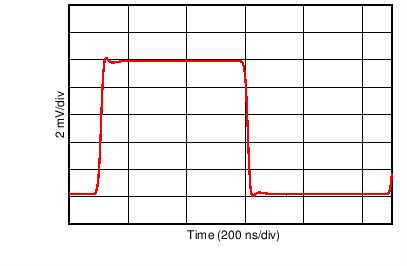10 mV, G = 1, CL = 10 pF
Figure 7-32 Small-Signal Step Response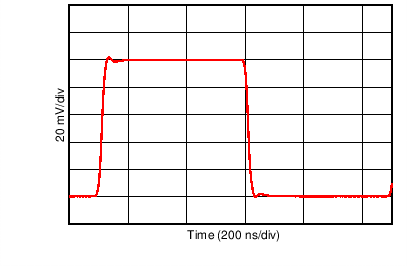100 mV, G = 1, CL = 10 pF
Figure 7-34 Small-Signal Step Response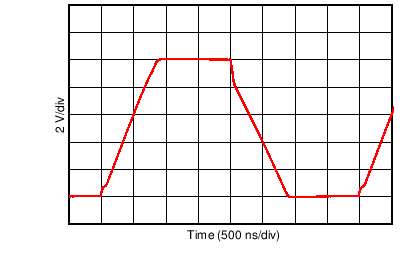10 V, G = 1, CL = 10 pF
Figure 7-36 Large-Signal Step Response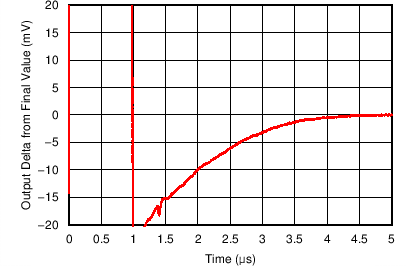G = 1, CL = 10 pF, 0.1% settling = ±10 mV
Figure 7-38 Large-Signal Settling Time (10‑V Negative Step)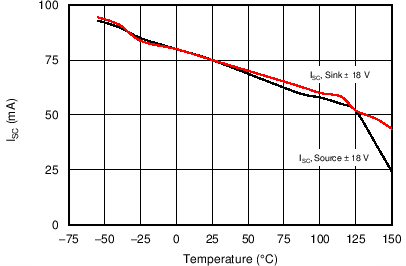Figure 7-40 Short-Circuit Current vs Temperature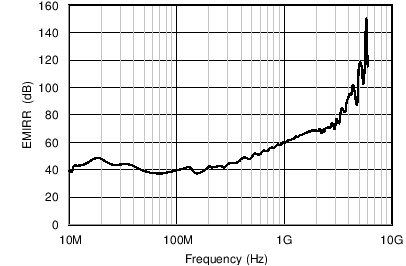PRF = –10 dBm, VSUPPLY = ±18 V, VCM = 0 V
Figure 7-42 EMIRR vs Frequency